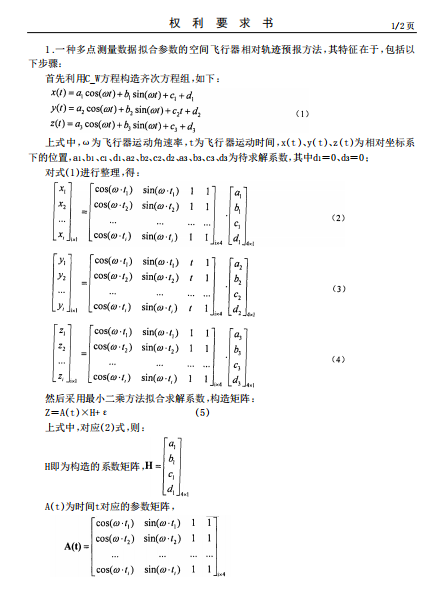010-68918340

知识成就未来

# 多点测量数据拟合参数的空间飞行器相对轨迹预报方法

Method for predicting relative orbits of space vehicles by making multi-point measurement data fit parameters

The invention belongs to the technical field of spacecraft dynamics and control and discloses a method for predicting relative orbits of space vehicles by making multi-point measurement data fit parameters. The method comprises the steps that a traditional dynamics-law-based C_W equation is converted into a system of homogeneous equations related to twelve constant coefficients, sines and cosines, the system of homogeneous equations are subjected to determinant conversion to construct a least square fit equation, the twelve constant coefficients are solved by accumulating the multi-point measurement data, the solved twelve constant coefficients serve as equation coefficients, and the relative orbits are predicted by time. By adopting the method, the influence of errors of a motion model and errors of an initial measurement value on prediction of the relative orbits can be remarkably overcome, and the prediction accuracy of the relative orbits of the space vehicles is improved.• 附件下载(1)：
•  2013180016858.pdf

北京理工大学国际交流中心812